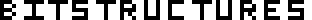## Functions, closures, and objects: implementing a filter function in Scheme and Java

Continuing with the theme of functions and closures (Registering JavaScript object methods as callbacks, Python bound methods), I thought I’d have a look at the relationship between functions, closures, and objects. I’ll build a filter, that returns elements from a collection for which a given predicate is true, in Scheme (a language with first-class functions and closures, but no objects), and in Java (a language with objects, but no first-class functions or closures.)

### A function is an object with an "apply" method

In Scheme we can define a filter function that takes two parameters: a function (the predicate), and a list. It will call the predicate function on each element in the list, returning a new list containing all of the elements for which the predicate is true:

``````(define (filter pred lis)
; if lis is empty
(if (null? lis)
; return an empty list
'()
; otherwise, if the predicate is true on the first element
(if (pred (car lis))
; return the first element concatenated with the
; result of calling filter on the rest of lis
(cons (car lis) (filter pred (cdr lis)))
; otherwise (if the predicate was false) just
; return the result of filtering the rest of lis
(filter pred (cdr lis)))))
``````

We can then define a predicate and use it to filter:

``````(define (non-negative n)
(>= n 0))

(filter non-negative '(-2 3 7 1)) ; (3 7 1)
(filter non-negative '(100 -9 8)) ; (100 8)
``````

In Java, we don’t have first-class functions, but we do have objects. And we can define a Predicate as an object with a public method that takes a single parameter and returns a boolean:

``````public interface Predicate<T> {
public boolean apply(T arg);
}
``````

We can now write our Java filter function as a method that takes two parameters: a Predicate and a Collection:

``````import java.util.Collection;
import java.util.List;
import java.util.ArrayList;

public class Utils {
public static <T> List<T> filter(Predicate<T> pred, Collection<T> c) {
List<T> filtered = new ArrayList<T>();
// loop through all the elements in c
for (T obj : c) {
// if the predicate is true for the current element
if (pred.apply(obj)) {
// append it to the result list
}
}
return filtered;
}
}
``````

NonNegative.java:

``````public class NonNegative implements Predicate<Integer> {
public boolean apply(Integer n) {
return n >= 0;
}
}
``````

With Predicate, Utils.filter, and NonNegative defined we can now filter:

``````// as our NonNegative class instances have no state,
// we only need one
Predicate<Integer> pred = new NonNegative();
Utils.filter(pred, Arrays.asList(-2, 3, 7, 1));
Utils.filter(pred, Arrays.asList(100, -9, 8));
``````

The Scheme and Java examples above operated on collections of numbers but the filter functions (and Predicate interface in the Java case) are generic – they can filter collections of any type and filter on any provided predicate logic.

### A closure is an object with an "apply" method and some state

Let’s say we want to filter all numbers greater than 4. We could define a greater-than-4 function:

``````(define (greater-than-4 n)
(> n 4))
``````

And if we wanted to filter all numbers greater than 1, we could define a function for that too. The greater-than-1 function is going to be very similar to greater-than-4 and it would be quite convenient to write a generic greater-than function:

``````(define (greater-than m n)
(> n m))

(greater-than 4 1) ; false
(greater-than 4 20) ; true
``````

Now, if we want to use our greater-than predicate with our filter function, we have a problem: the filter function expects the predicate to be a function that takes exactly one parameter (the element currently being considered), not two.

We can make our greater-than predicate work with our filter function by currying it:

``````(define (greater-than m)
; return a closure:
; a function that takes one parameter
; with a binding to the value provided for m
(lambda (n)
(> n m)))
``````

We have rewritten greater-than to be a builder of functions (that take one parameter, as we need for filter). Rather than calculating if n is greater than m immediately, we delay the test and package it up into a closure and return that. We provide the parameters m and n in two stages: m is provided when we call greater-than (and stored for later use in the closure) and n is provided whenever the function returned from greater-than is called. For example, if we wanted to calculate 1 > 4 and 20 > 4:

``````(define greater-than-4 (greater-than 4))
(greater-than-4 1) ; false
(greater-than-4 20) ; true
; or, without naming the function
((greater-than 4) 1) ; false
((greater-than 4) 20) ; true
``````

It is this two-stage quality that enables us to use greater-than with our filter function:

``````(filter (greater-than 4) '(-2 3 7 1)) ; (7)
(filter (greater-than 10) '(100 -9 8)) ; (100)
``````

We can do something very similar in Java, but rather than a closure we use an object. We can implement GreaterThan as a Predicate in which m is provided to the object constructor and stored in an instance variable. The apply method will retrieve it later to perform its test:

``````public class GreaterThan implements Predicate<Integer> {
private Integer m;
public GreaterThan(Integer m) {
this.m = m;
}
public boolean apply(Integer n) {
return n > m;
}
}
``````

No changes are necessary to either the Predicate interface or to Utils.filter:

``````Utils.filter(new GreaterThan(4), Arrays.asList(-2, 3, 7, 1));
Utils.filter(new GreaterThan(10), Arrays.asList(100, -9, 8));
``````